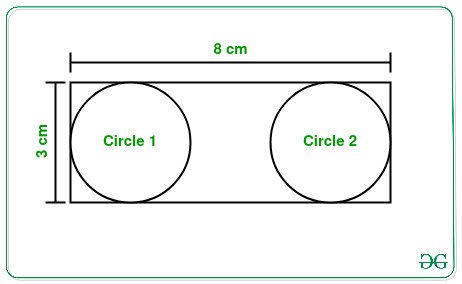GFG App
Open AppBrowser
Continue

# Number of largest circles that can be inscribed in a rectangle

Given two integers L and B representing the length and breadth of a rectangle, the task is to find the maximum number of largest possible circles that can be inscribed in the given rectangle without overlapping.

Examples:

Input: L = 3, B = 8
Output: 2
Explanation:From the above figure it can be clearly seen that the largest circle with a diameter of 3 cm can be inscribed in the given rectangle.
Therefore, the count of such circles is 2.

Input: L = 2, B = 9
Output: 4

Approach: The given problem can be solved based on the following observations:

• The largest circle that can be inscribed in a rectangle will have diameter equal to the smaller side of the rectangle.
• Therefore, the maximum number of such largest circles possible is equal to ( Length of the largest side ) / ( Length of the smallest side ).

Therefore, from the above observation, simply print the value of ( Length of the largest side ) / ( Length of the smallest side ) as the required result.

Below is the implementation of the above approach:

## C++

 `// C++ program for the above approach`   `#include ` `using` `namespace` `std;`   `// Function to count the number of` `// largest circles in a rectangle` `int` `totalCircles(``int` `L, ``int` `B)` `{` `    ``// If length exceeds breadth` `    ``if` `(L > B) {`   `        ``// Swap to reduce length` `        ``// to smaller than breadth` `        ``int` `temp = L;` `        ``L = B;` `        ``B = temp;` `    ``}`   `    ``// Return total count` `    ``// of circles inscribed` `    ``return` `B / L;` `}`   `// Driver Code` `int` `main()` `{` `    ``int` `L = 3;` `    ``int` `B = 8;` `    ``cout << totalCircles(L, B);`   `    ``return` `0;` `}`

## Java

 `// Java program for the above approach` `import` `java.io.*;` `import` `java.util.*;` `class` `GFG ` `{`   `  ``// Function to count the number of` `  ``// largest circles in a rectangle` `  ``static` `int` `totalCircles(``int` `L, ``int` `B)` `  ``{` `    ``// If length exceeds breadth` `    ``if` `(L > B) {`   `      ``// Swap to reduce length` `      ``// to smaller than breadth` `      ``int` `temp = L;` `      ``L = B;` `      ``B = temp;` `    ``}`   `    ``// Return total count` `    ``// of circles inscribed` `    ``return` `B / L;` `  ``}`   `  ``// Driver Code` `  ``public` `static` `void` `main(String[] args)` `  ``{` `    ``int` `L = ``3``;` `    ``int` `B = ``8``;` `    ``System.out.print(totalCircles(L, B));` `  ``}` `}`   `// This code is contributed by susmitakundugoaldanga.`

## Python3

 `# Python3 program for the above approach`   `# Function to count the number of` `# largest circles in a rectangle` `def` `totalCircles(L, B) :` `    `  `    ``# If length exceeds breadth` `    ``if` `(L > B) :`   `        ``# Swap to reduce length` `        ``# to smaller than breadth` `        ``temp ``=` `L` `        ``L ``=` `B` `        ``B ``=` `temp` `    `  `    ``# Return total count` `    ``# of circles inscribed` `    ``return` `B ``/``/` `L`   `# Driver Code` `L ``=` `3` `B ``=` `8` `print``(totalCircles(L, B))`   `# This code is contributed by splevel62.`

## C#

 `// C# program to implement` `// the above approach` `using` `System;` `public` `class` `GFG` `{`   `  ``// Function to count the number of` `  ``// largest circles in a rectangle` `  ``static` `int` `totalCircles(``int` `L, ``int` `B)` `  ``{` `    ``// If length exceeds breadth` `    ``if` `(L > B) {`   `      ``// Swap to reduce length` `      ``// to smaller than breadth` `      ``int` `temp = L;` `      ``L = B;` `      ``B = temp;` `    ``}`   `    ``// Return total count` `    ``// of circles inscribed` `    ``return` `B / L;` `  ``}`     `  ``// Driver Code` `  ``public` `static` `void` `Main(String[] args)` `  ``{` `    ``int` `L = 3;` `    ``int` `B = 8;` `    ``Console.Write(totalCircles(L, B));` `  ``}` `}`   `// This code is contributed by souravghosh0416.`

## Javascript

 ``

Output:

`2`

Time Complexity: O(1)
Auxiliary Space: O(1)

My Personal Notes arrow_drop_up